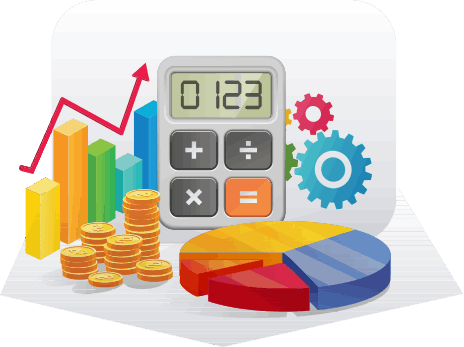html templates#### Calculators

Compound interest refers to interest that is gained on the total of principal (i.e. the initial amount invested) plus any interest that was previously earned in the account.

For example, you invest \$10,000 for two years at an annual interest rate of 5%.At the end of the first year, the interest accrued (earned) will be \$500 (i.e. 5% of \$10,000) and the balance in your account at the end of the first year would be \$10,500 (i.e. \$10,000 plus \$500).

At the end of the second year, the interest accrued would be \$525 (i.e. 5% of \$10, 500) and the balance in your account at the end of the second year would be \$11,025 (i.e. (\$10,500 plus interest of \$525).

The above example demonstrates that the longer you leave money in an account, the greater potential it has to to grow and allow you to earn you more money. For each year that money is left in an account, the annual interest earned will be on a growing principal sum.

The key factor in the accrual of compound interest is TIME.
Use the Compound Interest Annuity Calculator below to illustrate the benefits of compounding, especially for a long period of time.